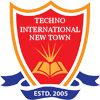# Control System Laboratory-I

PC-EE 593 (5th Semester)

Major Equipments and Software:

• Hardware Kit for synchro Transmitter & Receiver with position Control.
• Process control Simulator (for linear system & PID Study).
• Dual Trace Digital storage Oscilloscope.
• DC Motor Speed and Position Control system with Analog PID controller
• Digital controller based on Arduino and computer Interfacing.
• MATLAB

Experiment List:

• Familiarization with MAT-Lab control system toolbox, MAT-Lab- Simulink toolbox
• Determination of Step response for first order & Second order system with unity feedback with the help of CRO & calculation of control system specification, Time constant, % peak overshoot, settling time etc. from the response.
• Simulation of Step response & Impulse response for type-0, type-1 & Type-2 system with unity feedback using MATLAB
• Determination of Root locus, Bodeplot, Nyquist plot using MATLAB control system toolbox for a given system & stability by determining control system specification from the plot.
• Determination of PI, PD and PID controller action of first order simulated process.
• Determination of approximate transfer functions experimentally from Bode plot.
• Study of a practical position control system obtaining closed step responses for gain setting corresponding to overdamped and under-damped responses. Determination of rise time and peak time using individualized components by simulation. Determination of un-damped natural frequency and damping ratio from experimental data.
• Analysis of performance of Lead, Lag and Lead-Lag compensation circuits for a given system using simulation.
• Determination of Transfer Function of a given system from State Variable model and vice versa.
• Analysis of performance of a physical system using State variable technique by simulation. Study of step response and initial condition response for a single input, two-output system in SV form by simulation.

• Study of a practical position control system obtaining closed step responses for gain setting corresponding to overdamped and under-damped responses. Determination of rise time and peak time using individualized components by simulation. Determination of un-dampednatural frequency and damping ratio from experimentaldata.

• Tuning of P, PI and PID controller for first order plant with dead time using Z-N method. Process parameters (time constant and delay/lag) will be provided. The gain of the controller to be computed by using Z-N method. Steady state and transient performance of the closed loop plant to be noted with and without steady disturbances. The theoretical phase margin and gain margin to be calculated manually for each gain setting.

• Design of Lead, Lag and Lead-Lag compensation circuit for the given plant transfer function. Analyze step response of the system by simulation.

• Obtain Transfer Function of a given system from State Variable model and vice versa. State variable analysis of a physical system - obtain step response for the system by simulation.

• State variable analysis using simulation tools. To obtain step response and initial condition response for a single input, two-output system in SV form by simulation.

• Performance analysis of a discrete time system using simulation tools. Study of closed response of a continuous system with a digital controller and sample and hold circuit by simulation.

• Study of the effects of nonlinearity in a feedback controlled system using time response. Determination of step response with a limiter nonlinearity introduced into the forward path of 2nd order unity feedback control systems. The open loop plant will have one pole at the origin and other pole will be in LHP or RHP. To verify that

(i) with open loop stable pole, the response is slowed down for larger amplitude input
(ii) for unstable plant, the closed loop system may become oscillatory with large input amplitude by simulation

•   Study of effect of nonlinearity in a feedback controlled system using phase plane plots. Determination of phase plane trajectory and possibility of limit cycle of common nonlinearities.

Beyond the Curriculum:

• Demonstration of real-time position control system with PC based motor control control setup.
• Demonstration of tuning of P, PI and PID controller for PC based position control system.